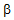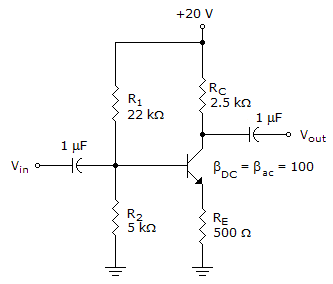# Electronic Devices - BJT Amplifiers

### Exercise :: BJT Amplifiers - General Questions

6.

Which of the following conditions must be met to allow the use of the approximate approach in a voltage-divider bias configuration?

 A.re > 10R2 B.RE > 10R2 C.RE < 10R2 D.re < 10R2

Explanation:

No answer description available for this question. Let us discuss.

7.

Refer to this figure. Determine the value of Av.A. 49.6 B. 5 C. 100 D. 595

Explanation:

No answer description available for this question. Let us discuss.

8.

For a common-emitter amplifier, the purpose of swamping is

 A. to minimize gain. B. to reduce the effects of r'e C. to maximize gain. D. no purpose.

Explanation:

No answer description available for this question. Let us discuss.

9.

What is the typical value of the current gain of a common-base configuration?

 A. Less than 1 B. Between 1 and 50 C. Between 100 and 200 D. Undefined

Explanation:

No answer description available for this question. Let us discuss.

10.

What is the most important r parameter for amplifier analysis?

 A. rb′ B. rc′ C. re′